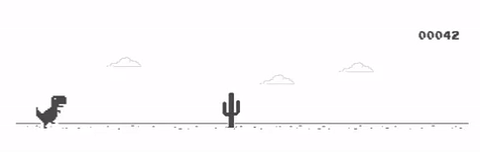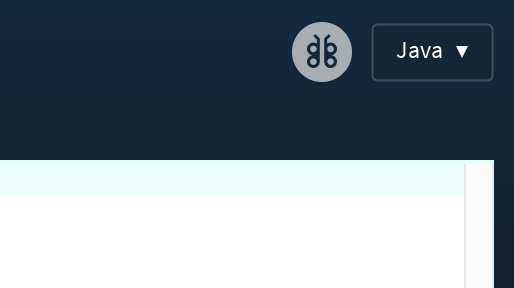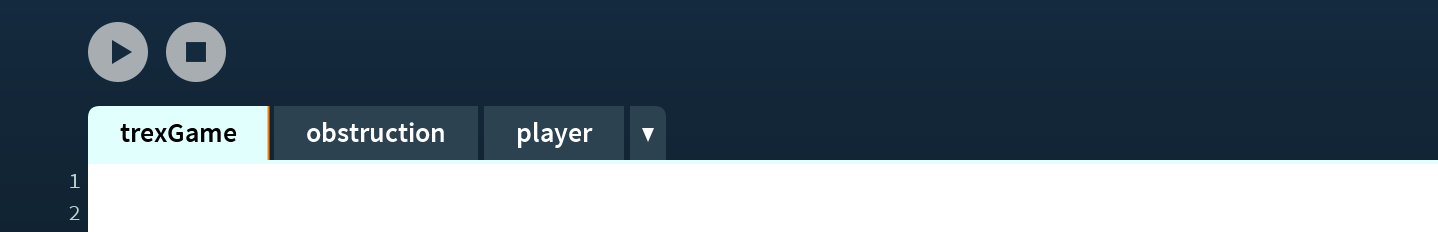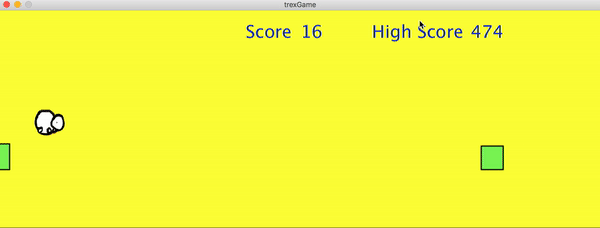×

Search anything:

# Chrome Dinosaur (T-Rex 🦖) game with Processing in Java

#### Software Engineering JavaGet this book -> Problems on Array: For Interviews and Competitive Programming

We all have played the t-rex game on chrome! Its time to make our own now.We will be using Processing for the same, and you may install it at https://processing.org/download/.

Make sure you have opened it in Java mode.Our game consists of the following entities,

1. The player (the T-rex)
2. The Obstructions

We may create 2 more files (tabs in the processing window) for the above entities. These are actually `.pde` files.Each entity has some associations in the final game, described as follows. They are well interweaved, and so we will go step-by-step building the game and identifying the association.

#### Canvas

We first need somewhere to actuall play the game. Since its related to the overall game, we will be setting up our canvas in `trexGame.pde`.
It requires a `setup` function, to draw the canvas of predefined size, looking somethin like this.

``````void setup()
{
size(1100,400); //Initialise a canvas
}
``````

Everything on this canvas, is drawn by means of a `draw` function in the same file. We have chosen a pale yellow colour for this background, and the function looks something like this.

``````void draw()
{
background(255,255,102);
}
``````

#### Make the player

We shall start with putting the player in place and making it jump! We must declare a class for the player in `player.pde`. Within it we require the following data members:

• A position vector
• An Acceleration vector for upward and downward acceleration for jumping
• A Velocity vector

Since these are vectors and thus have an x and y value, we will initialise them as `PVector` type.

We will be using a circle to represent our player and so need a data member of type float to keep the radius.

We must initialise these data members in the constructor. Feel free to experiment with initialising values. Our class should currently look like this.

``````class Player{

PVector pos; //This contains position of player
PVector acc; //This contains acceleration of player
PVector vel;  //This contains velocity of player

float r=40; ////This contains radius of player object

Player()
{
//initialise the player data members
pos = new PVector(50,(height-200));
vel = new PVector(0, 20);
acc = new PVector();
}
};
``````

Then we need some functions to use our player.
We shall start with displaying our player, and shall declare a `show` function. This function should have `void` return type. To display, we use:

• The `fill` function which takes RGBA value of colour as input and fills the subsequent object.
• The `stroke` function which takes RGBA value of border-colour of object.
• The `strokeWeight` function which takes the weight/thickness of border in pixels.
• The `ellipse` function to make the circle. Circle is also a type of ellipse and hence is created using this function. It takes, x-coordinate, y-coordinate, length of major axis and length of minor axis as parameters.

Your show function must look something like this.

``````void show()
{
fill(255,0,34);
stroke(0,0,0);
strokeWeight(2);
ellipse( pos.x,pos.y,r*2,r*2);

}
``````

We wish to use our logo's snowball instead of the circle, and so will make the following changes.

• In `trexGame.pde` initialise a variable as `PImage pl;` to contain the image.
• Load the image in the variable in the setup function as `pl = loadImage("snowball.png");`.Here "snowball.png" is actually the Relative Path to the image
• In the `show` function of `Player` replace `ellipse( pos.x,pos.y,r*2,r*2);` with
``````imageMode(CORNER);
image(pl, pos.x,pos.y,r*2,r*2);
``````

wherein `imageMode` reflects where exactly is the image drawn on the object. feel free to experiment with other image modes, and `image` displays the image stored in the first argument.

The position shall also be constantly updated for which we will require an update function. Before this, we will need the following:

• We need an `applyForce` function in order to apply force. This is required as we will be applying both upward and downward forces to jump, and so we we may rather declare a function for it as follows:
``````void applyForce(PVector force)
{
}
``````
• We will apply an upward force for it to jump, but what will pull it back down then? Gravity! hence, we need to define gravity. Since gravity is a property of the system we will do this in `trexGame.pde` as `PVector gravity = new PVector(0, 0.1);` on top.

Now lets create an update function. This shall also have `void` return type. We shall go about it as follows:

• First we need to apply gravity, as gravity is constantly acting upon us, by writing `applyForce(gravity);`.
• Now we shall update the position as `pos.add(vel);`.
• The position shall be limited such that it doesn't go beyond the frame. We shall do that by stating a condition that if the player goes below a certain point, we reduce the velocity to 0 and y position, by the following if statement:
``````if(pos.y >=height-170)
{
pos.y=height-170;
vel.mult(0);
}
``````
• Update the velocity according to acceleration using `vel.add(acc);`.
• For a smooth run we are capping the velocity by saying `vel.limit(4);`, you are free to experiment with this.
• After every update, I reduce the acceleration to zero by writing `acc.mult(0);`, since we are reapplying it, this ensures that the acceleration's value is in control. Try removing it and see what happens, later.

Your update function must look like:

``````void update()
{
applyForce(gravity); //applies downward gravity
pos.add(vel); // in order to update pos wrt velocity
if(pos.y >=height-170)
{
pos.y=height-170;
vel.mult(0);
}

vel.add(acc); //in order to update the vel as per acc
vel.limit(4); // in order to cap the vel for a smooth run

acc.mult(0);
}
``````

That is it for the player, we shall now move on to the barriers. In `barrier.pde` create a class Barrier. It shall contain the following data members:

• A float value for the height of the barrier.
• A float value for the width of the barrier.
• A float value to contain the x-coordinate of the obstructions.
• A float value to keep track of rate of appearance of the barriers.

Initialising values, I have given the bottom height a random value, just for fun! You can do whatever you like. In the constructor, increment x according to width of screen so that the location updates in different frames. For that make a variable `wid` in `trexGame.pde` as it is an attribute of the overall game (Canvas).

``````int wid = 1100; //The width of our screen
``````

Your class should look like this right now.

``````class Barrier
{
float bottom; //This contains height of barriers
float w = 40; //This contains width of barriers
float x; //This contains x location of the barrier
float barrier_speed = 3; //This is the rate of change in position of barrier

Barrier()
{
bottom = random(150, 160); //Set value of height of barrier
x = wid + w; //Increase the x location by with
}
``````

We are maintaining a start variable to make sure that the game is running. The barriers only come up if the game is running. So go to `trexGame.pde` and declare a variable as:

``````boolean start=false; //Keeps track of whether the game is going on
``````

Now in the barrier class we first need an `update` function to update the x-coordinate of the barriers according to `barrier_speed`. We do that as follows

``````void update ()
{
//if the game is going on modify barrier x-locations if the game is going on
if(start)
{
x -= barrier_speed;
}
}
``````

We also need to know the the player collides with the barrier. We shall Declare a function named `hits` with `boolean` return type taking the Player object `b` as parameter. We check:

• If the x-coordinate falls in the width of the barrier by `((b.pos.x > x)&&(b.pos.x < (x+wid)))`
• If the y-coordinate falls across the diameter of the circle by `((b.pos.y < (top + b.r)) || (b.pos.y > (height - bottom - b.r)))`

These are joined using && i.e. if both stated conditions are true, only then the statement evaluates as true else it is false. Hence the function looks like:

``````//Check for collision, if locations of the player and the pipe is overla
boolean hits(Player b)
{
return ((b.pos.x > x) && (b.pos.x < (x + wid))) &&  (b.pos.y > (height - bottom - b.r));
}
``````

Next, we need to display our barriers, here we will change the colour of the barrier on a hit. So the `show` function will take a boolean parameter `hit` telling whether the player hit the barrier.

• If the game is in progress (`start` is True).
• If the Barrier is hit (`hit` is True).
• We fill with this Red colour by `fill(217,74,30,127);`
• Else
• We fill with this Green colour by `fill(65,224,129,127);`
• We give a black border with weight of 2 pixels.
• We create a rectangle at position (`x` , `height - bottom`) where `height` contains height of the canvas of width `w` and height `bottom` by writing `rect(x, height - bottom, w, bottom-110);`

The function must look like:

``````void show(boolean hit)
{

if(start) //display barriers if game is in progress
{
if(hit)
{
//fill red if we hit the barrier
fill(217,74,30,127);
}
else
{
//fill green normally
fill(65,224,129,127);
}
stroke(0,0,0);
strokeWeight(2);
rect(x, height - bottom, w, bottom-110);

}

}
``````

Now it is time to bring all this action in `trexGame.pde`.

• Define a `Player b;` on top to play with.
• This player needs to
• since there will be multiple varying barriers, we will be using an `ArrayList` for the same, define that as `ArrayList<Barrier> barriers = new ArrayList<Barrier>();`

In the draw function
We want to display are barriers only if the game is progressing. So we shall write the following within `if (start) {}`.

We want:

• Barriers to appear randomly
• Barriers to appear at a playable distance

So, for playable distance we may ensure distance in terms of frames, i.e.`frameCount % 60 == 0`.
For randomness we may give appearance roughly 50% probability by saying `random(1)<0.5`.
Feel free to experiment with these values.

We must add a new Barrier then. So the code will look like

``````if(start)
{
//Add barriers at random distances such that
//minimum distance is 60 frames to make the
//game playable only if th game is in progress.
if(random(1)<0.5&&frameCount % 60 == 0)
{
}
}
``````

Pressing they key plays an important role.

• It starts the game.
• It makes the player jump

So if the key is pressed we shall

• Start the game by making `start=true;`
• Declare and apply an upward force, only when the player is at ground.

This shall look like:

``````if(keyPressed)
{
start = true; //Start the game on pressing the key
if(b.pos.y == height-170) //Jump only if the player is already on the ground
{
PVector up = new PVector(0,-100); //Defining an appropriate upward force
b.applyForce(up); //Applying the upward force just defined
}

}
``````

Now we can start displaying!
First comes the player, so you may update and show the player by writing:

``````b.update(); //Update the player's position and speed
b.show(); //Display the player
``````

Now we need to display the barriers. such that we check whether the bird hits the barrier and pass the `boolean hit` in show function accordingly.

``````for(int i=barriers.size()-1; i>=0; i--)
{
Barrier p = barriers.get(i);
p.update();
p.show(p.hits(b));
}
``````

We can also remove the barriers from the list that are no lnger in frame by adding the following in the for loop.

``````if(p.x < -p.w)
{
barriers.remove(i);
}
``````

Our game must be functioning by now, we can display the score and high score, along with a message which tells CLICK TO START

For this we will declare the following data members:

• `boolean safe=true;` to know whether the player has hit a barrier or not.
• `int score=0;` to keep track of current score.
• `int highScore=0;` to keep track of high score.

Now we may modify the statement in the barrier's display for loop such that when `p.hits(b)` is not only sent in the `show` function but also updates the variable `safe`.

It should now look like

``````for(int i=barriers.size()-1; i>=0; i--)
{
Barrier p = barriers.get(i);
p.update();

if (p.hits(b))
{
p.show(true);
safe=false;
}
else
{
p.show(false);
safe=true;
}

//Remove the barriers that went out of frame
if(p.x < -p.w)
{
barriers.remove(i);
}
}
``````

If the game goes on smoothly, update score, or else restart the game. Restarting includes

• Display CLICK TO START
• Set `start=false` since game now needs to be started on a click
• Set `score=0`.

This can be done as follows

``````  if(safe&&start) //Increment the score if game is going on smoothly
{
score++;
}
else
{
//Restart the game
score=0;
text("CLICK TO START",width/2-500,50);
start=false;
``````

To display the score we need to

• Fill the score text with colour by `fill(16,53,201);`
• Set size of the text by writing `textSize(32);` where 32px is size of text.
• Display the text as follows, where `width/2-100` and `width/2` are x-coordinates and `50` is y-coordinate for text.
``````text("Score",width/2-100,50);
text(score,width/2,50);
``````

Similarily Set and display high score

``````//Set and display high score
if(highScore < score)
{
highScore = score;
}

text(highScore,width/2+310,50);
text("High Score",width/2+300-170,50);
``````

And that is it!! This is how it looks:Go to https://github.com/OpenGenus/t-rex for the complete code! You are welcome to build your own version now!! cheers!#### Priyanshi Sharma

Priyanshi Sharma has been an Intern at Google in 2021 | Computer Science student at National Institute of Technology, Raipur | 2020 Intern at OpenGenus | Author of "Problems in AI" |

Chrome Dinosaur (T-Rex 🦖) game with Processing in Java